Next:Information and Divergence MeasuresUp:Entropy of a Continuous

Entropy-Power Inequality

Given a continuous random variable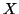with differential entropy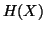, the entropy power ofis given by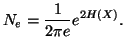We have the following two properties.

Property 1.77. Letand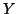be independent continuous random variables of finite variance. Then the differential entropy satisfies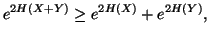(1.14)

with equality iffandare gaussian.

Property 1.78. Let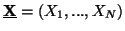and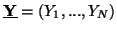be two independent continuous vector valued random variables with finite variance. Then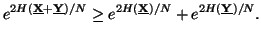Note 1.8. The inequality (1.14) is famous in the literature as "Power inequality". Some studies on it can be seen in Stam (1959) , Blachman (1965)  and Costa (1985) .

21-06-2001
Inder Jeet Taneja
Departamento de Matemática - UFSC
88.040-900 Florianópolis, SC - Brazil